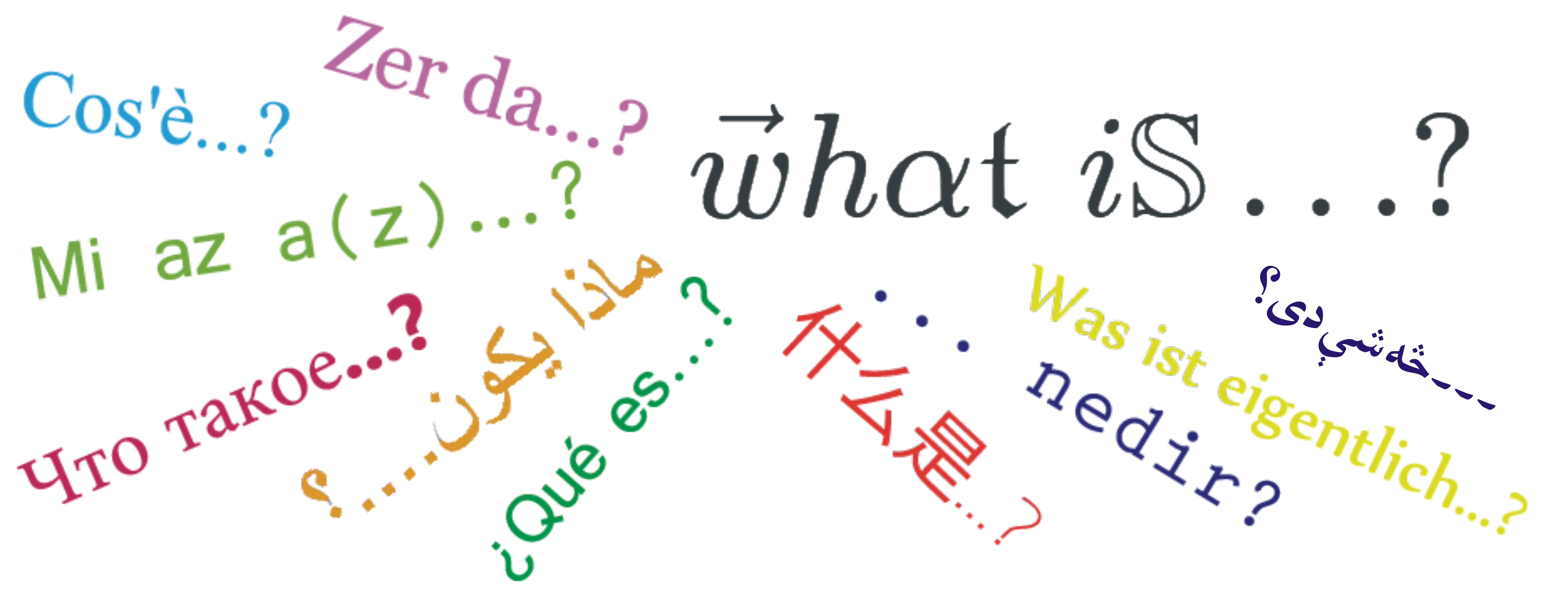## What is the $\vec{w}h\alpha\mathfrak{t}\;\; i\mathbb{S}\ldots\text{?''}$ Seminar?

The $\vec{w}h\alpha\mathfrak{t}\;\; i\mathbb{S}\ldots\text{?''}$ Seminar is a cycle of talks organized each semester by students at the three universities of Berlin, the Freie Universität Berlin, the Humboldt-Universität zu Berlin and the Technische Universität Berlin. Each talk of the $\vec{w}h\alpha\mathfrak{t}\;\; i\mathbb{S}\ldots\text{?''}$ Seminar is characterized by the following:

1. It lasts around half an hour.
2. It introduces a mathematical idea or object answering a question of the form “What is...?”.
3. It is directed to mathematics students or mathematicians unfamiliar with the exposed field.
4. It happens in an open and relaxed environment where comments and questions are welcome.

## When is the $\vec{w}h\alpha\mathfrak{t}\;\; i\mathbb{S}\ldots\text{?''}$ Seminar?

The $\vec{w}h\alpha\mathfrak{t}\;\; i\mathbb{S}\ldots\text{?''}$ Seminar takes place almost every Friday of each of the semesters either as a prelude to a MATH+ Friday Colloquium or as a stand-alone talk by a student wanting to speak a particular topic. The talks usually take place at 13:00, although there can be variations for those sessions that happen on a Friday without a colloquium.

## Where is the $\vec{w}h\alpha\mathfrak{t}\;\;i\mathbb{S}\ldots\text{?''}$ Seminar taking place?

When the seminar takes place in person, there are four primary locations:

However, the talks can take place in other places on exceptional dates.

## Support

The $\vec{w}h\alpha\mathfrak{t}\;\; i\mathbb{S}\ldots\text{?''}$ Seminar receives the support of the

which is the the graduate school of mathematics of the three univeristies of Berlin, Freie Universität Berlin, Humboldt-Universität zu Berlin and Technische Universität Berlin. The BMS provides the $\vec{w}h\alpha\mathfrak{t}\;\; i\mathbb{S}\ldots\text{?''}$ Seminar with video equipment, seminar rooms and funding for several events.